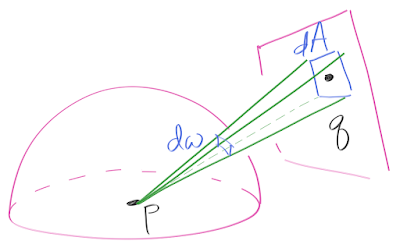## Thursday, March 31, 2016

### Converting area-based pdf to direction-based pdf

One thing I covered in my new mini-book is dealing with pdf management in a path tracer.     Most of my ray tracers (and many others do it too) are set up to sample directions.   So at a point being shaded, the integral is over directions:

color(direction_out) = INTEGRAL   brdf(direction_in, direction_out) cosine

the domain of integration is all direction_in coming from the sphere of all directions.   Often the brdf is zero for directions from inside the surface so it's the hemisphere.

Basic Monte Carlo integration says that if you choose a random direction_in with pdf pdf(direction_in), the unbiased estimate is:

color(direction_out)  =  brdf(direction_in, direction_out) cosine / pdf(direction_in)

As long as you can generate random direction_in, and can compute pdf(direction_in) then you have a working ray tracer.   It works best when you are likely to cast random rays toward bright areas like lights.    If you sample a polygonal light, you normally just sample q uniformly on the area.   The direction is just q-p.    The key observation to get from the pdf in area space p_area(q) = 1/A (where A is the area of the light) is that by definition the probability of q being in a small area dA is

probability = p_area(q) dA

Similarly the directional pdf of a direction toward dA is:

probability = pdf(q-p) dw

Those probabilities must be the same:

p_area(q) dA =  pdf(q-p) dw

By algebra:

pdf(q-p) =  p_area(q) dA/dw = (1/A) (dA/dw)

But what is (dA/dw)?

Let's do some basic geometry.   As is so often the case, the right figure makes that tons easier:You pick a point q on the light with pdf 1/A in area space, but what is the pdf in directional space?   Drawn in limnu whitboarding program.

If dA faces p dead-on (so its normal faces p), then it's foreshotening:

dw = dA/distance_squared

But if dA is tilted then we get a cosine shift:

dw = dA*cosine / distance_squared

So recall we are after

pdf(q-p) = (1/A) (dA/dw)

So we can plug and chug to get:

pdf(q-p) =length_sqaured(q-p) / (A*cosine)

We just apply this formula directly in our code.   For my xz rectangle class this is: